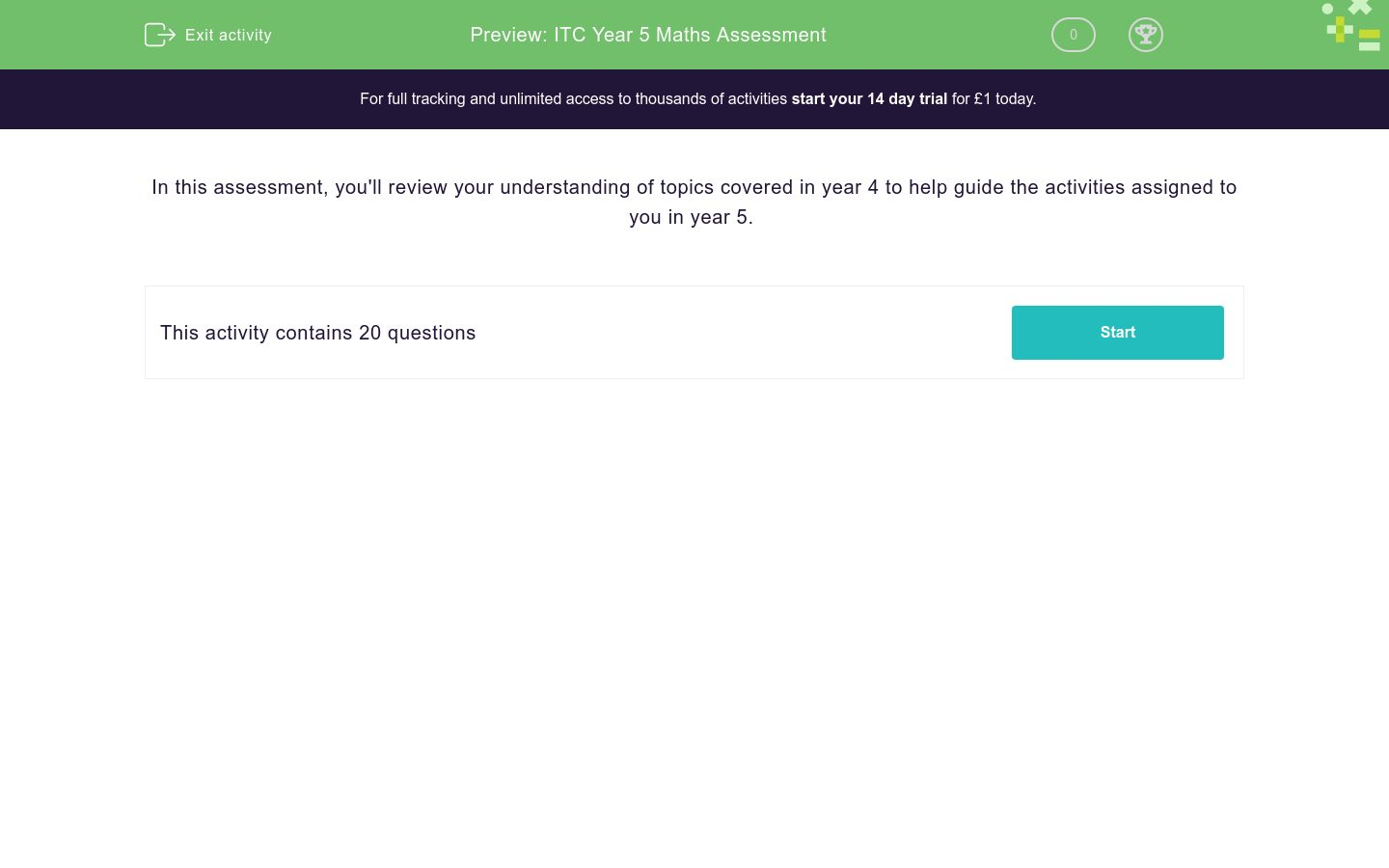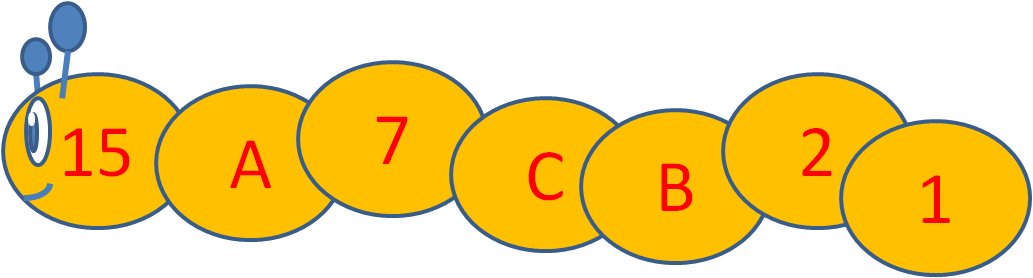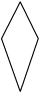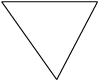# ITC Year 5 Maths Assessment

In this assessment, students will review their comprehension of topics covered at the end of year 4 to help guide their activities and progress in year 5.Key stage:  KS 2

Curriculum topic:   Assessment

Curriculum subtopic:   Starter Assessment

Difficulty level:### QUESTION 1 of 10

In this assessment, you'll review your understanding of topics covered in year 4 to help guide the activities assigned to you in year 5.

Choose < or > to place between these pairs of numbers.

201 -163
>

<

If the number is even, divide it by 2.

If the number is odd, subtract 1 from it.

Stop when you get to the number 1.

Work out the values of A, B and C in this number worm, using the rule above.## Column B

A
3
B
6
C
14

Subtract the numbers.

769 - 99 = ?

We know that 620 - 436 = 184.

Which other facts can we get from this?

436 + 184 = 620

436 - 184 = 620

184 + 436 = 620

184 + 620 = 436

620 - 184 = 436

Find the number that makes the sum correct.

13 + __ = 200

Tick all the factor pairs of:

108

1 and 108

9 and 12

2 and 54

3 and 36

4 and 27

6 and 18

Work out

253 × 5

What is... ?

81 ÷ 9

Use the factor pair 8 × 7 = 56 to work out

560 ÷ 7

Count up in hundredths to work out the missing number.30.5    30.51    30.52

What is the missing number?

 + 4 . 8 6 2 . 5 2 1 . 8 3

Write the missing number.

 3 + 15 = 18 24 24 ?

Use the number line to help you convert this fraction into a decimal amount.

75¾34
×  12  =

Each of Amir's four children get £4.30 pocket money every week.

How much does this cost Amir in pounds every week?

Just write the number.

﻿Calculate the area of this shape, made of centimetre squares and half squares.What is the length of one side of this regular pentagon in cm?Perimeter: 20 cm

Which of these shapes are not right-angled triangles?abcdefgh
a

b

c

d

e

f

g

h

Look at this regular star (pentagram).

Which of the lines are lines of symmetry?a

b

c

d

e

The table below shows the number of different coloured cars that drove past my house between Monday and Wednesday.

How many more grey cars than red cars passed in total over Monday and Wednesday?• Question 1

Choose < or > to place between these pairs of numbers.

201 -163
>
EDDIE SAYS
Positive numbers are all bigger than negative numbers.
• Question 2

If the number is even, divide it by 2.

If the number is odd, subtract 1 from it.

Stop when you get to the number 1.

Work out the values of A, B and C in this number worm, using the rule above.## Column B

A
14
B
3
C
6
EDDIE SAYS
15 is odd, so subtract 1 to get A.
7 is odd, so subtract 1 to get C.
Halve C to get B, since C is even.
• Question 3

Subtract the numbers.

769 - 99 = ?

670
EDDIE SAYS
Subtract 100 and then add 1 back.
• Question 4

We know that 620 - 436 = 184.

Which other facts can we get from this?

436 + 184 = 620
184 + 436 = 620
620 - 184 = 436
EDDIE SAYS
Adding is the opposite of subtracting.
Remember that we can add in reverse too.
• Question 5

Find the number that makes the sum correct.

13 + __ = 200

187
EDDIE SAYS
Work out 200 - 13.
• Question 6

Tick all the factor pairs of:

108

1 and 108
9 and 12
2 and 54
3 and 36
4 and 27
6 and 18
EDDIE SAYS
108 = 1 × 108 = 2 × 54 = 3 × 36 = 4 × 27 = 6 × 18 = 9 × 12
• Question 7

Work out

253 × 5

1265
EDDIE SAYS• Question 8

What is... ?

81 ÷ 9

9
EDDIE SAYS
9 × 9 = 81
• Question 9

Use the factor pair 8 × 7 = 56 to work out

560 ÷ 7

80
EDDIE SAYS
8 × 7 = 56
80 × 7 = 560
560 ÷ 7 = 80
• Question 10

Count up in hundredths to work out the missing number.30.5    30.51    30.52

What is the missing number?

30.49
EDDIE SAYS
30.49... 30.5 or 30.50... 30.51... 30.52
• Question 11

 + 4 . 8 6 2 . 5 2 1 . 8 3
9.21
EDDIE SAYS
Add in columns, starting at the right. Carry if the answer is 10 or more. Keep the decimal points lined up.
• Question 12

Write the missing number.

 3 + 15 = 18 24 24 ?
24
EDDIE SAYS
24ths added to 24ths stay as 24ths.
• Question 13

Use the number line to help you convert this fraction into a decimal amount.

75¾75.75
• Question 14
 34
×  12  =
9
EDDIE SAYS
¼ of 12 = 12 ÷ 4 = 3
Multiply this by 3 to get ¾ of 12.
• Question 15

Each of Amir's four children get £4.30 pocket money every week.

How much does this cost Amir in pounds every week?

Just write the number.

17.20
17.2
EDDIE SAYS
£4.30 × 4 = £17.20
• Question 16

﻿Calculate the area of this shape, made of centimetre squares and half squares.9
EDDIE SAYS
There are 2 × 4 = 8 whole centimetre squares.
There are also 2 half-squares.
8 + 1 = 9
• Question 17

What is the length of one side of this regular pentagon in cm?Perimeter: 20 cm
4cm
4 cm
4
EDDIE SAYS
20 ÷ 5 = 4
• Question 18

Which of these shapes are not right-angled triangles?abcdefgh
a
b
c
d
e
g
EDDIE SAYS
f and h are triangles with right angles inside.
• Question 19

Look at this regular star (pentagram).

Which of the lines are lines of symmetry?a
b
c
d
e
EDDIE SAYS
A regular pentagram has 5 lines of symmetry from each point to the opposite 'indent'.
• Question 20

The table below shows the number of different coloured cars that drove past my house between Monday and Wednesday.

How many more grey cars than red cars passed in total over Monday and Wednesday?20
EDDIE SAYS
(24 + 33) - (21 + 16) = 57 - 37 = 20
---- OR ----

Sign up for a £1 trial so you can track and measure your child's progress on this activity.

### What is EdPlace?

We're your National Curriculum aligned online education content provider helping each child succeed in English, maths and science from year 1 to GCSE. With an EdPlace account you’ll be able to track and measure progress, helping each child achieve their best. We build confidence and attainment by personalising each child’s learning at a level that suits them.

Get started